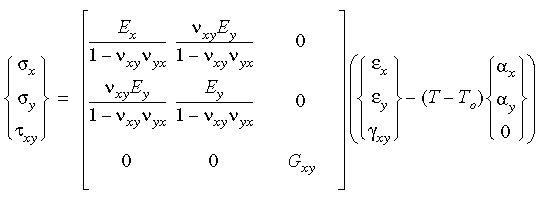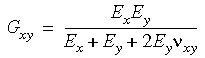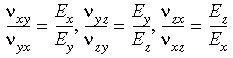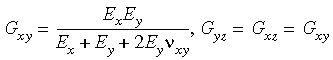# Linear Elastic Orthotropic Model

In contrast to an isotropic material, an orthotropic material has preferred directions of strength which are mutually perpendicular. The properties along these directions (also known as principal directions) are the extreme values of elastic coefficients.

The [D] matrix for an orthotropic material has nine independent elastic properties. In addition, there are three properties for the thermal expansion.

## 2-D Orthotropic Stress-Strain Relations

In two dimensions, the orthotropic stress-strain relations can be written as follows, including temperature effects:Note that in order to satisfy symmetry in the matrix of elastic moduli, νxy Ey = νyx Ex.

You need to satisfy the foregoing symmetry condition when you input the numerical values of either the elastic modulus or Poisson's ratio.

Further, if you do not input the numerical value of the shear modulus, the program will compute it as shown below:In three dimensions, the orthotropic symmetry conditions dictate:When you input the orthotropic material properties in three dimensions, you must therefore make sure that the above symmetry conditions are not violated.

Note that if you do not input the numerical values of shear moduli, the program will compute them using the relations shown below:If Ex = Ey = Ez, the program calculates the shear moduli internally even if explicitly defined. The program assumes 0.0 for Poisson's ratios that are not explicitly defined.